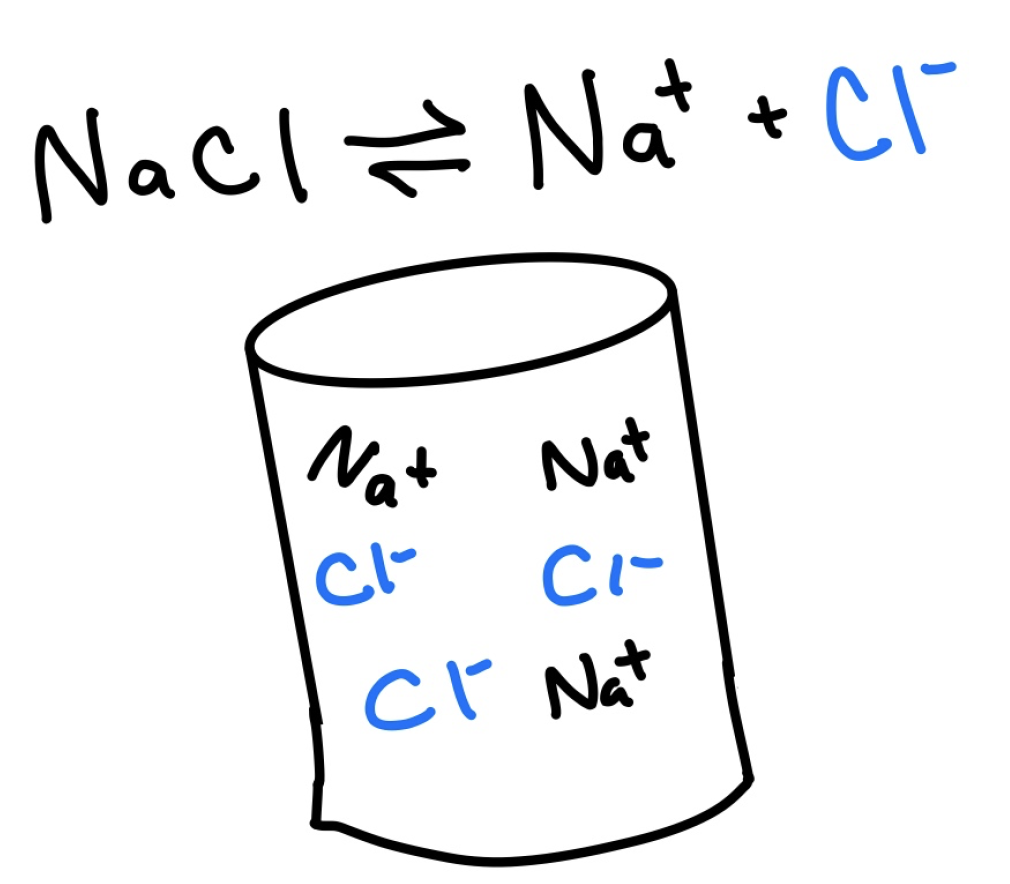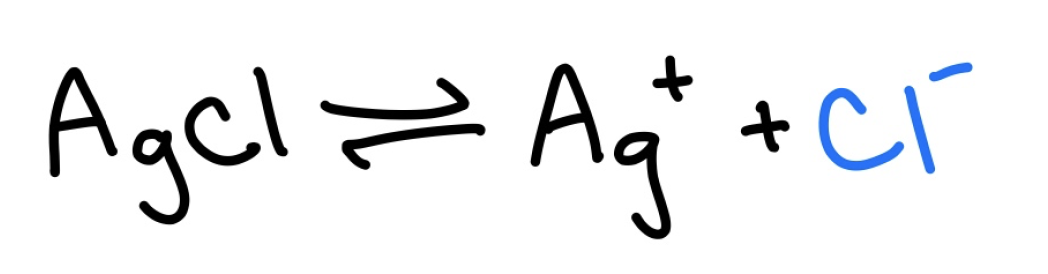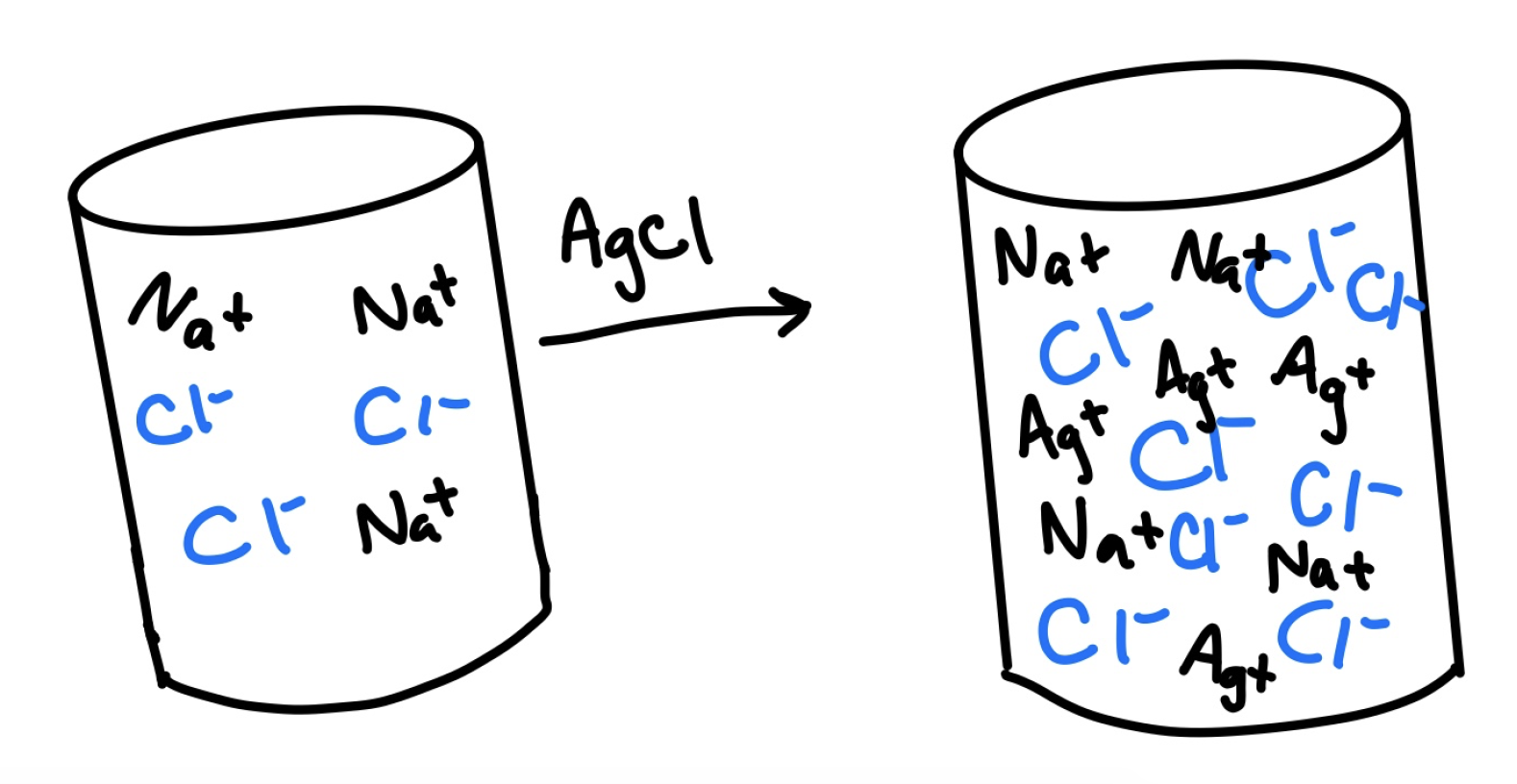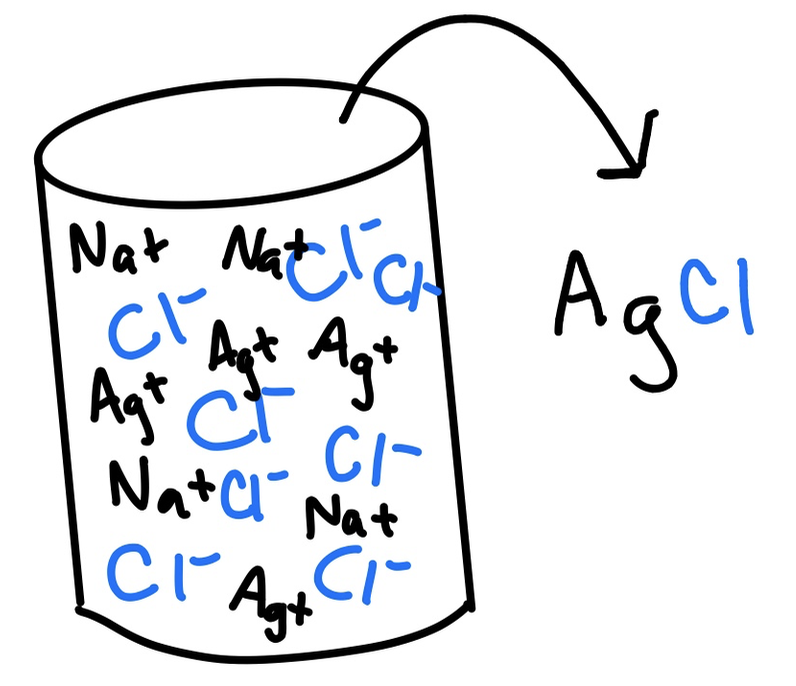Blog / Common Ion Effect And Its Use In Laboratory Separations

### Common Ion Effect and Its Use in Laboratory Separations

Written by Sabina Cabrera on Feb 12, 2020

The common ion effect is the decrease in solubility (ability to be dissolved) of a substance through the addition of another substance with a common ion; this effect is attributed to the shift in equilibrium.

In an equilibrium equation, there are two sides with chemical species known as the products (right) and reactants (left). In this case of the equilibrium equation of sodium chloride, NaCl, the products would be Na+ and Cl, and the reactant would be solid NaCl. The common ion effect is the visual result seen from shifting the equilibrium; a shift in equilibrium results in the creation of either more products or reactants being created.For example, let us say we fully dissolved table salt, NaCl, in a glass of water, leaving no solid NaCl left in solution:

There are now both Na+ and Cl– ions in the solution, as seen in the equilibrium equation. If we were to dissolve silver chloride, AgCl, in the same glass, the equilibrium equation would look something like this:However, because NaCl and AgCl both contain Cl ions, the equilibrium of the reaction shifts to the left. This can be explained by Le Chatlier’s Principle, which dictates the equilibrium of a reaction to shift in whichever direction is needed to counteract the effect. In this situation, the effect is the surplus of the reactant Cl. As a result, because the reaction favors the left side, AgCl, its solubility is decreased.As seen in the figure above, NaCl and AgCl dissolve to form Na+, Ag+, and Cl ions. Because NaCl and AgCl share the common ion Cl, all Cl ions in solution contribute to the equilibrium of AgCl.In laboratory experiments, we can use the common ion effect to crash out specific compounds in a solution. For example, if we have silver chloride, AgCl, and silver sulfate, Ag2SO4, in solution and wanted to only take out AgCl, we could think about our example above and add NaCl to crash out the AgCl in solution.Key Points:

• The common ion effect occurs when an ionic compound (a substance that contains ions) comes into contact with a substance sharing a common ion and decreases the solubility of the ionic compound.

Key Terms:

• Ionic: substance composed of ions
• Equilibrium: State in which reactions and products are in a state that does not have a tendency to change over time.
• Le Chatlier’s Principle: Explains that changes in the temperature, volume, concentration, and pressure will shift the equilibrium in a predictable manner.
• Product: End result of a reaction; the ride side of an equilibrium equation.
• Reactant: Combined with other reactants to form the product; the left side of an equilibrium equation.

Billing Information